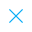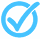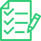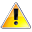Call Support +91-85588-96644

Keep me logged in
You can't leave Captcha Code empty
By submitting this form, you agree to the Terms & Privacy Policy.
ORTests given

OR# Probability - IX (Level 2)

Questions

Time

Highest
score

Level

## ENGLISH

Language

Topics Covered:Calculating Empirical ProbabilityCalculating Empirical ProbabilityCalculating Empirical ProbabilityCalculating Empirical ProbabilityCalculating Empirical ProbabilityCalculating Empirical ProbabilityCalculating Empirical ProbabilityCalculating Empirical ProbabilityCalculating Empirical ProbabilityCalculating Empirical Probability

### Latest Tests#### Surface Areas and Volumes - III (Class IX)

20 Min - 12 Ques

Take Test#### Areas of Parallelograms and Triangles (Class - IX)

20 Min - 15 Ques

Take Test#### Polynomials (Set - I)

15 Min - 12 Ques

Take TestThis is a concept-building practice test and may not have exact structure as you would expect in the actual exam. Please exercise your discretion to attempt it or go to structured Featured Section.

Description:
Probability IX (Level 2)
 Type Comment Question

## Personalised Learning Plans

Class X
Topper(s) of this Test
•### Preety

Score: 11/12

•### Manpreet Singh

Score: 6.5/12Related Tests